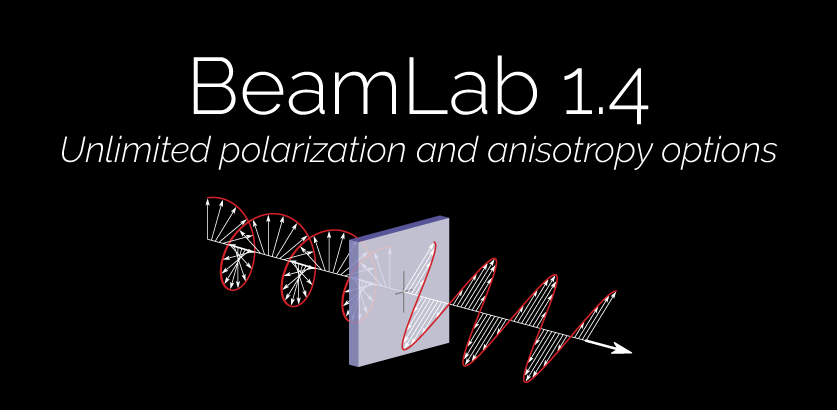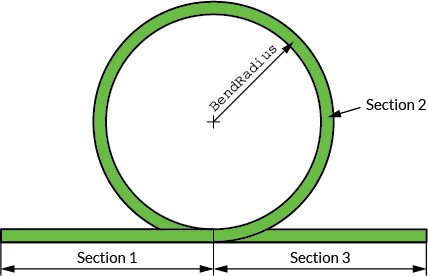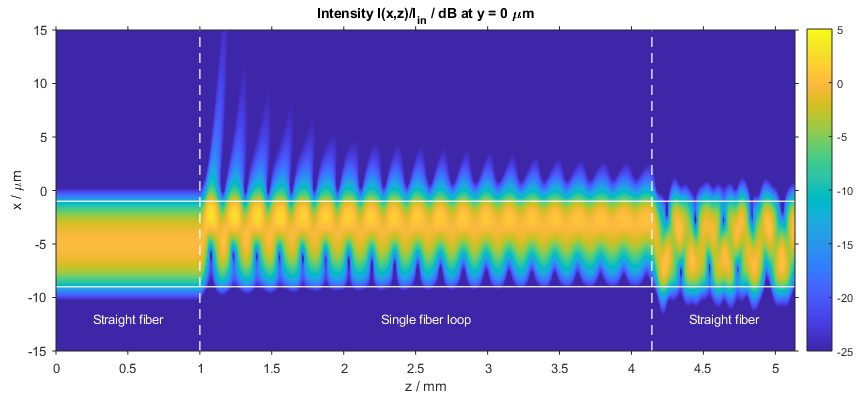# BeamLab 1.4Say hi to BeamLab 1.4 — another major release with some amazing new features and enhancements! This time we enhanced BeamLab to work with any input polarization state and a wide variety of anisotropic materials. Read on to learn what’s new (jump to release notes).

# Arbitrary input polarizations

Beginning with this release, it is now possible to define arbitrary polarization states of input fields via a polarization vector that corresponds to the normalized Stokes vector [S1 S2 S3] with S0 = 1. When using multiple input fields, the `Polarization` vectors can even be defined independently from each other. For example, the following demo shows the behavior of two Gaussian beams when they cross each other in free space — in one case they have the same circular polarization defined by a `Polarization` vector of `[0 0 1]` and thus strongly interfere with each other, while in the other case they are orthogonally polarized with `Polarization` vectors of `[0 0 1]` and `[0 0 -1]` and do not interfere at all. With BeamLab’s simple user interface, all it takes to define these input fields is two lines of MATLAB® code:

Case 1 (same circular polarization):

```inputField{1} = @(b) gaussinput(b,[30 30],'Shift',[ 30 0 0],'Angle',[-5 0],'Polarization',[0 0 1]);
inputField{2} = @(b) gaussinput(b,[30 30],'Shift',[-30 0 0],'Angle',[ 5 0],'Polarization',[0 0 1]);```

Case 2 (orthogonal circular polarization):

```inputField{1} = @(b) gaussinput(b,[30 30],'Shift',[ 30 0 0],'Angle',[-5 0],'Polarization',[0 0 1]);
inputField{2} = @(b) gaussinput(b,[30 30],'Shift',[-30 0 0],'Angle',[ 5 0],'Polarization',[0 0 -1]);```

The following video shows the beam propagation of the two different sets of input fields:

Video of the propagation of two Gaussian beams with the same (top) and orthogonal (bottom) polarizations

# Analysis of bent waveguides

We’ve also added the possibility to calculate a virtual bend in `singlecore` and `rib` waveguides. The following demo shows how the fundamental mode propagating in a silica fiber with a core index delta of 2% starts to radiate light off the core if configured as a fiber loop with a bending radius of 0.5 mm. The waveguide structure consists of 3 sections where the fiber loop (section 2) with a total length of 3.14 mm was inserted between two straight fiber sections each having a length of 1 mm.Schematic of the fiber loop

Video of the intensity profile of the beam propagating in the fiber loopIntensity distribution in the fiber loop (xz slice)

# Propagation through anisotropic media

The possibility of defining arbitrary polarization states at the input makes it also interesting to study the change of a light wave’s polarization state when propagating through birefringent media. In the following demo, a circularly polarized Gaussian beam is first sent through a quarter-wave plate transforming the circular input polarization into a linear output polarization with the polarization plane tilted by 45°. After a short length of free-space propagation, a half-wave plate then rotates the polarization plane further from 45° to 90°, i.e., parallel to the y-axis. While the quarter-wave plate’s two principal axes are oriented in parallel to the x– and y-axis, the principle axes of the half-wave plate have been rotated by 67.5° in counter-clockwise direction to achieve the additional rotation of the polarization plane by 45°. Both wave plates have a basic refractive index of 1.5 and a thickness of 10 µm requiring a relative birefringence of 1% and 2% for the quarter-wave and half-wave plate, respectively. The wavelength used in this simulation is 0.6 µm. The whole propagation structure with five sections is defined by the following simple MATLAB® code:

```indexFunction{1} = @(b) homogeneous(b,2,1);
indexFunction{2} = @(b) homogeneous(b,10,1.5,'Anisotropy',[1 1.01 1]);
indexFunction{3} = @(b) homogeneous(b,2,1);
indexFunction{4} = @(b) homogeneous(b,10,1.5,'Anisotropy',[1 1.02 1],'Rotation',67.5);
indexFunction{5} = @(b) homogeneous(b,2,1);```

Video of the beam propagating through a quarter-wave and a half-wave plate

Interested in more examples? Please also check out our examples page.

# Release notes

### New features:

• Add the possibility to define the input polarization via a polarization vector (i.e., normalized Stokes vector) that allows one to work with arbitrary input polarizations. In this respect, the `Polarization` parameter has been moved from `beamset` to the input field functions. Both semi-vectorial and full-vectorial BPM calculations can be performed with arbitrary input polarizations. For semi-vectorial mode calculations the desired polarization can be set via the new parameter `ModePolarization`.
• Add information on the E-field’s polarization state at the x-y slices and output plane to the output data structure of a BPM calculation.
• Add the possibility to calculate a virtual bend in `singlecore` and `rib` waveguides.
• Add possibility to define the anisotropy of waveguides by means of a 3- or 5-element vector representing the relative components `[xx,yy,zz]` or `[xx,yy,zz,xy,yx]` of the index tensor.
• Add new optional parameters `AnisotropyCore`, `AnisotropySubstrate`, and `AnisotropyCladding` to `rib`, replacing the single parameter `Anisotropy`. This allows one to define the refractive index anisotropy for core, substrate, and cladding individually.
• Add two new options `'DvsLambda'` and `'DvsV'` to the parameter `DispersionGraphType` for plotting the dispersion parameter D as a function of wavelength and normalized frequency, respectively.
• Add scale options `'lincustom'` and `'logcustom'` to parameters `SlicesXYScale`, `SlicesXZScale`, `SlicesYZScale`, and `PowerTraceScale`. The new scale options allow one to define a linear or logarithmic scale, respectively, normalized to a custom value. In case of `SlicesXYScale`, `SlicesXZScale`, and `SlicesYZScale`, this custom value is defined by the new optional parameter `SlicesCustomNorm` and in case of `PowerTraceScale`, the custom value is defined by the newly added parameter `PowerCustomNorm`.
• Add possibility to address simultaneously an arbitrary ensemble of cores with `ModeCore` and thus calculate the (super)modes defined by these cores.
• Add new optional parameter `ModeSelect` that allows one to select specific modes (either in form of a scalar or a vector) out of a larger mode ensemble.
• Add the possibility to define `modesolver` relevant parameters such as `ModeCore`, `ModeNumber`, `ModePolarization`, `ModeSections`, `ModeSelect`, and `ModeSortingOrder` directly as input parameters of `modeinput`. In addition, `modeinput` now also allows one to define an input distribution that consists of a linear combination of modes with different mode amplitudes by assigning a vector containing the relevant mode numbers to `ModeSelect` and a vector containing the appropriate mode amplitudes to `ModeAmplitude`.
• Add new optional parameter `Phase` to all input field functions for specifying the input field’s phase in degrees.
• Add possibility to display phase and index distributions in x-z and y-z slices.
• Add possibility to display multiple x-z and y-z slice plots of different types.
• Add new optional parameter `QuiverLength` that allows one to adjust the length of the quiver arrows.
• Add new optional parameter `ShowSectionTitles` for displaying section titles inside the figure during monitor. The position inside the figure can be specified by the new parameter `SectionTitlePosition`.
• Add option to `beamlabdoc` for searching the documentation for pages with words matching a specified expression.
• Add demo `fiber_loop`, which simulates the propagation of the fundamental mode of a fiber through a fiber loop.
• Add demo `fiber_bend_modes`, which calculates the first three eigenmodes of a fiber with a bending radius of 10 cm.
• Add demo `fiber_bend_loss`, which calculates the bending loss of the first three eigenmodes of a fiber with a bending radius of 10 cm as a function of the wavelength.
• Add demo `quarterwave_plate`, which simulates the propagation of a Gaussian beam with circular polarization through a quarter-wave plate.
• Add demo `noninterfering_beams`, which simulates the propagation of two orthogonally polarized Gaussian beams that cross each other in free space.
• Add demo `fiber_taper`, which simulates the propagation of the fundamental mode of a fiber whose size is changed by means of a fiber taper.

### Enhancements:

• Eliminate the need for the user-defined parameters `FieldSymmetryX` and `FieldSymmetryY`. Field symmetries are now set automatically according to the input field and index symmetries `SymmetryX` and `SymmetryY`.
• Extend full-vectorial `bpmsolver` to work also with anisotropic `homogeneous` and `singlecore` waveguides that are rotated by some arbitrary angle.
• Improve power area definition. The `SmoothingLevel` parameter is now also used for smoothing the rim of the power area.
• Improve display of quiver arrows in x-y slice plots.
• Improve performance of license manager.
• Improve demo scripts.
• Improve documentation.

### Bug fixes:

• Fix bug in `dispersionsolver` when using cores with index trenches.
• Fix bug in `indexplot` when using `fasthomogeneous` sections.
• Fix bug when modeling a tapered `rib` waveguide.
• Fix license manager bug when using macOS or Linux.
• Various minor bug fixes.